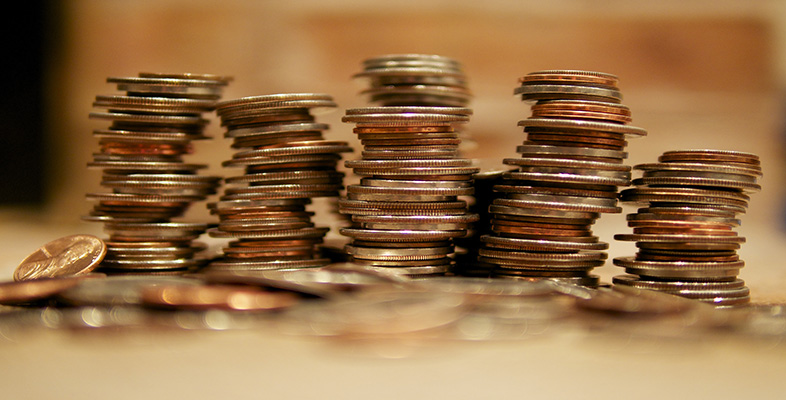Start this free course now. Just create an account and sign in. Enrol and complete the course for a free statement of participation or digital badge if available.

Free course

# 1.3 The arithmetic mean

Another important measure of location is the arithmetic mean. (Pronounced arithmetic.)

## Arithmetic mean

The arithmetic mean is the sum of all the values in the batch divided by the size of the batch. More briefly,There are other kinds of mean, such as the geometric mean and the harmonic mean, but in this course we shall be using only the arithmetic mean; the word mean will therefore normally be used for arithmetic mean.

## Example 4 An arithmetic mean

Suppose we have a batch consisting of five values: 4, 8, 4, 2, 9. In this simple example, the mean isNote that in calculating the mean, the order in which the values are summed is irrelevant.

For a larger batch size, you may find it helpful to set out your calculations systematically in a table. However, in practice the raw data are usually fed directly into a computer or calculator. In general, it is a good idea to check your calculations by reworking them. If possible, use a different method in the reworking; for example, you could sum the numbers in the opposite order.

The formula ‘’ can be expressed more concisely as follows. Referring to the values in the batch by, the ‘sum’ can be written as. Hereis the Greek (capital) letter Sigma, the Greek version of S, and is used in statistics to denote ‘the sum of’. Also, the symbolis often used to denote the mean – and as you have already seen in stemplots,can be used to denote the batch size. (Some calculators use keys markedandto produce the sum and the mean of a batch directly.)

Using this notation,can be written asIn this course we shall normally round the mean to one more figure than the original data.

## Activity 3 Small televisions: the mean

The prices of 20 small televisions were given in Activity 1 (Subsection 1.2). Find the mean of these prices. Round your answer appropriately (if necessary), given that the original data were rounded to the nearest £10.

### Discussion

Using the data for the prices from Activity 1:Or using thenotation,and, soThe prices were rounded to the nearest £10, so it is appropriate to keep one more significant figure for the mean, that is, to show it accurate to the nearest £1. So since the exact value is £162, it needs no further rounding.

M140_1